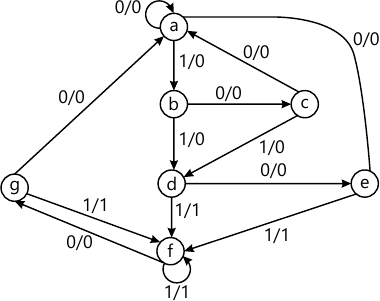MORE IN Digital Electronics
Total marks: --
Total time: --
INSTRUCTIONS
(1) Assume appropriate data and state your reasons
(2) Marks are given to the right of every question
(3) Draw neat diagrams wherever necessary

Answer any one question from Q1 and Q2
1 (a) Explain following characteristics of digital ICs
1. Noise margin
2. Fan in & Fan out
2 M
1 (b) Simplify and implement following expression using K-map.
Y=∑m (1,5,6,7,11,12,13,15)
4 M

2 (a) Draw CMOS circuit for NOR gate.
2 M
2 (b) Design and implement following function using 4:1 multiplexer F=∑m(1,3,4,5).
4 M

Answer any one question from Q3 and Q4
3 (a) Draw and explain TTL to CMOS interface.
4 M
3 (b) What do you mean by multiplexer tree? Explain.
2 M

4 (a) Give comparisons between TTL, ECL and CMOS logic families.
4 M
4 (b) What do you mean by priority encoder?
2 M

Answer any one question from Q5 and Q6
5 (a) Draw and explain SR Flip Flop using NAND gates.
2 M
5 (b) Convert D to T flipflop.
4 M

6 (a) What is clock skew and clock jittering in synchronous circuits?
2 M
6 (b) Design a mod-6 synchronous counter.
4 M

Answer any one question from Q7 and Q8
7 (a) Compare Mealy machine with Moore machines.
2 M
7 (b) Design a sequence detector to detect the sequence 110, using JK flip-flops. Use Mealy Machine.
4 M

8 Reduce following state diagram.6 M

Answer any one question from Q9 and Q10
9 (a) Draw and explain CPLD with its block diagram.
6 M
9 (b) Design seven-segment decoder using PLA.
7 M

10 (a) Differentiate between static and dynamic RAM?
4 M
10 (b) Compare between different types of PLDs.
3 M
10 (c) Implement following function using PLA.
F1 (A, B, C) = ∑M (0, 2, 5, 7)
F2 (A, B, C) = ∑M (2, 3, 4, 5)
6 M

Answer any one question from Q11 and Q12
11 (a) Differentiate between signals and variables.
4 M
11 (b) What is a structural type of modelling? Explain with an example.
4 M
11 (c) Write VHDL code for 3:8 decoder using case statement.
5 M

12 (a) Explain architecture with different modelling styles.
6 M
12 (b) Explain the difference between concurrent and sequential statements.
4 M
12 (c) Explain loop statement with example.
3 M

More question papers from Digital Electronics